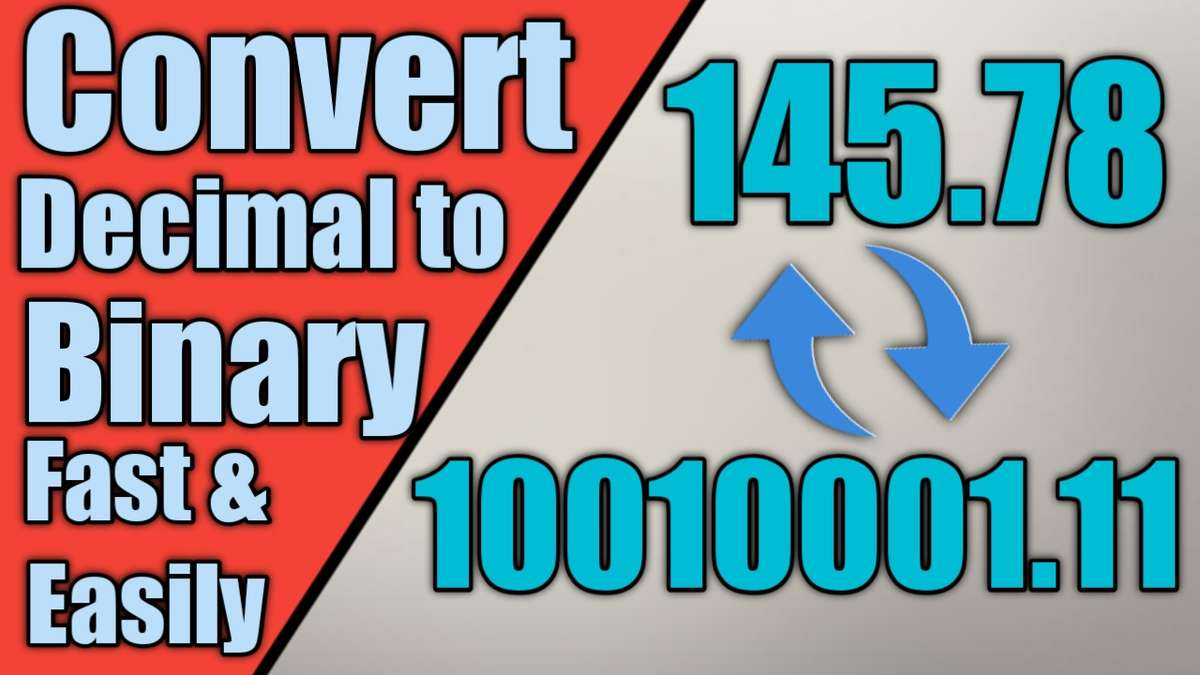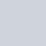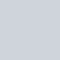# Easy and fast way to convert Decimal to Binary and Binary to Hexadecimal or OctalPublished

on

#### Hello Friends Welcome In My Blog And Today I Share With You A Easy To understand fast trick to convert decimal to binary and binary to decimal and hexadecimal or octal

Let We want To convert 263 from decimal to binary so First Type Some Numbers starting from right side with the relation of every number is double from previous number like This :  256  128  64  32  16  8  4  2  1

And now we need to find the highest number which is under/equal 263 and 256 is highest number under/equal 263 and we right 1 under 256 and we need 7 to complete 263 and find the highest number under/equal 7 and 4 is highest number under 7 and right 1 under 4  and now we need 3 to complete 263.

And again find the highest number under/equal 3 and 2 is highest number right 1 under 2 and now we need 1 to complete 263 and again find highest number under/equal 1 and 1 is highest number under/equal 1 and right 1 under 1 and right 0 under all numbers which is not used to complete 263 And After You can see you got binary number of 263 is 100000111